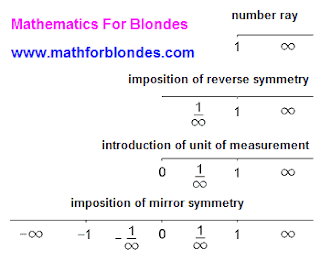## 9/27/2011

### Number line

In mathematics it is accepted to represent numbers as a number line. We will consider transformation of number ray to the number line.

Reverse symmetry allows to get numbers less unit. As a point of reverse symmetry is unit, this symmetry does not depend on units of measurement. Reverse symmetry reflects relativity of concepts "greater than unit" and "less than unit" at comparison of two numbers. In case of comparison of two numbers without fail it is needed to accept one of these numbers as unit of measurement.

After introduction of unit of measurement we get the absolute system of coordinates for any unit of measurement. Unit of measurement on a picture is represented in the traditionally accepted variant - with imposition on the area of reverse numbers. At imposition of mirror symmetry the point of that is a zero, we enter negative numbers and get the relative system of coordinates. All enumerated transformations are represented on a picture below, where the sign of endlessness is mark any number.Number line

Expl for blondes: Farther we will consider relativity of concept "Any number". More interesting things on the page "New Math".